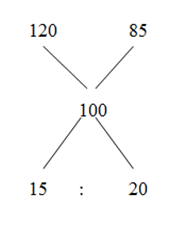# gkrecall

We brings you daily Current affairs, daily Current Affairs Quiz, weekly Current Affairs, weekly Current Affairs Quiz to enhance your preparation for upcoming exams. We also provide articles related to quant, reasoning, English, ssc etc .

# Arithmetic sum for RRB PO/CLERK AND RBI ASSISTANT MAINS

1) Raju is as much older than Moni as he is younger than Anu. If the sum of the ages of Anu and Moni is 56 years, then find the present age of Raju?

a) 28 years

b) 24 years

c) 22 years

d) 26 years

e) None of these

2) Find the difference between the compound interest on Rs. 30000 for 2 years at 10 % per annum compounded yearly and half yearly?

a) Rs. 172.225

b) Rs. 184.625

c) Rs. 136.5175

d) Rs. 165.1875

e) None of these

3) A man purchases 2 watches for Rs. 665. He sells one of them at 20% profit and another at 15% loss. Thus, he neither gains nor loses. Find the cost price of both the watches?

a) Rs. 342, Rs. 323

b) Rs. 285, Rs. 380

c) Rs. 410, Rs. 353

d) Rs. 267, Rs. 323

e) None of these

4) A and B invest in the ratio of 12 : 9. If they divide 30 % of the profit equally and rest in the proportion of their investments, then find the profit of B if A’s profit is Rs. 2090?

a) Rs. 1650

b) Rs. 1710

c) Rs. 1520

d) Rs. 1400

e) None of these

5) The marked price of an item is Rs. 3200 more than the cost price. When the discount Rs. 400 is allowed, the profit 20 % is earned. At what price should the item be sold to earn the profit of 25 %?

a) Rs. 16250

b) Rs. 17500

c) Rs. 21750

d) Rs. 23800

e) None of these

6.  A person invests money in 3 different schemes for 3 years, 5 years and 6 years at 10%, 12% and 15% simple interest respectively. At the completion of each scheme, he gets the same interest. The ratio of his investments is:

A. 4:3:3

B. 3:2:1

C. 6:3:2

D. 5:2:4

E. 1:1:2

7. A sells a horse to B for Rs. 9720, thereby losing 19 per cent, B sells it to C at a price which would have given A 17 per cent profit. Find B’s gain.

A. 4220

B. 4400

C. 4000

D. 4141

E. 4320

8. In an election between 2 candidates, 75% of the voters cast their votes, out of which 2% votes were declared invalid. A candidate got 18522 votes which were 75% of the valid votes. The total number of voters enrolled in the election was:

A. 28200

B. 31300

C. 24500

D. 33600

E. 30000

9. The length of each side of a rhombus is equal to the length of the side of square whose diagonal is 80√2. If the length of the diagonals of rhombus are in ratio 3:4, then its area (in cm^2) is:

A. 6144

B. 6424

C. 6901

D. 5580

E. 6000

10. Two vessels A and B contains milk and water mixed in the ratio 8:5 and 5:2 respectively. The ratio in which these two mixture be mixed to get a new mixture containing 69(3/13)% milk is:

A. 3:4

B. 1:3

C. 2:7

D. 7:8

E. 8:9

Raju – Moni = Anu – Raju

2* Raju = Anu + Moni

Sum of the ages of Moni and Anu = 56 years

2*Raju = 56

Raju = 56/2 = 28 years

Compound interest = P (1 + R/100)N – P                 (where P = principal amount, R = rate of interest,  N = time)

Compounded yearly,

= > 30000 * (110/100) * (110/100) = 36300

C.I = 36300 – 30000 = Rs. 6300

Compounded half yearly,

= > 30000 * (105/00) * (105/100) * (105/100) * (105/100) = 36465.1875

C.I = 36465.1875 – 30000 = Rs. 6465.1875

Required difference = 6465.1875 – 6300 = Rs. 165.1875

CP1 + CP2 = 665= > 3 : 4

7’s = 665

1’s = 95

CP1 = 95*3 = 285

CP2 = 95*4 = 380

Let the total profit be x,

A’s profit = (15/100) * x + (70/100) * x * (12/21)

2090 = (15/100) * x + (40/100) * x

(55/100) * x = 2090

X = 2090*(20/11) = Rs. 3800

B’s profit = 3800 – 2090 = Rs. 1710

Let the C.P be x,

M.P = 3200 + C.P = 3200 + x

1. P = (3200 + x) – 400 = 2800 + x

S.P = (120/100) * x = (6/5) * x

2800 + x = (6/5) * x

2800 = (1/5) * x

C.P (x) = 14000

To earn a profit of 25 %,

P = (125/100)*14000 = Rs. 17500

6. Option C

(3×10%)of A = (5 × 12%) of B = (6 × 15%) of C

(A, B, C are the investments)

0.3 A = 0.6 B = 0.9 C

A:B:C: = 6:3:2

7. Option E

CP for A = 9720*100/(100-19) = 12000

SP with 17% profit for A = 12000*(100+17)/100 = 14040

B’s gain = 14040 – 9720 = 4320

8. Option D

Let total number of voters = x

Voters who cast their votes = 0.75x

Valid votes polled for a candidate

=> 18522 = 0.98*0.75*0.75x

=>x = 33600

9. Option A

Length of each side of rhombus = 80 cm

Let the diagonals = 3x, 4x

(3x/2 )^2 + (4x/2 )^2 = 6400

X^2 = 6400 ×4/25 = 1024

=> x = 32

Area of rhombus = 1/2× 96 × 128 = 6144 cm^2

10. Option C

8/13 ==== 5/7

====9/13

2/91 ====1/13

2:7